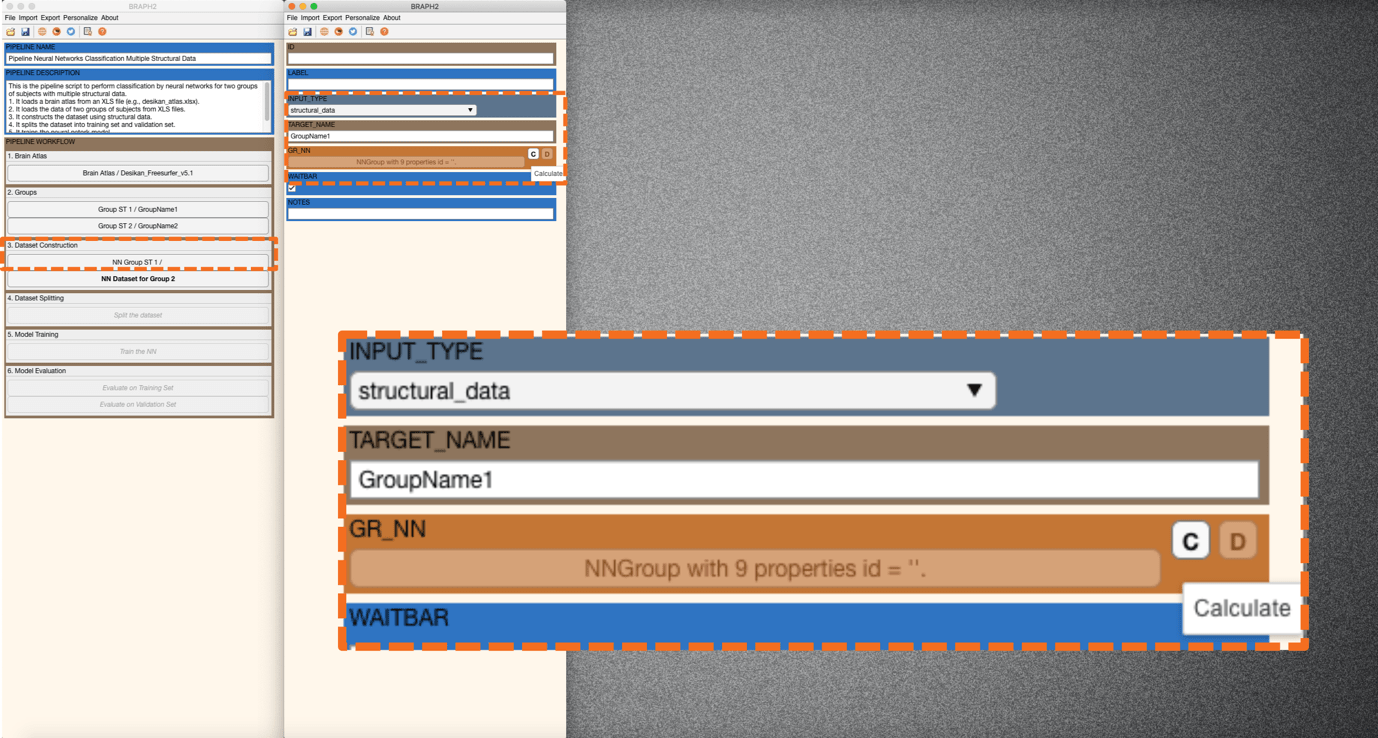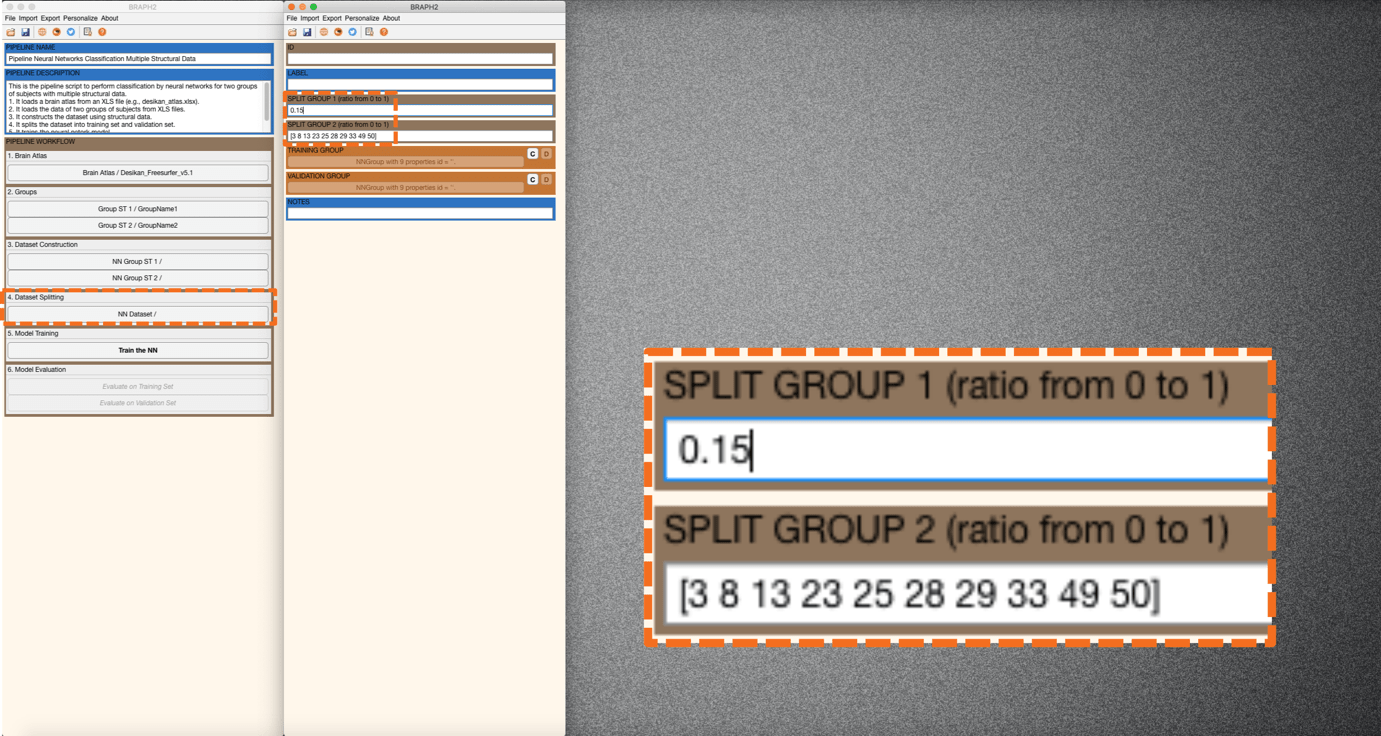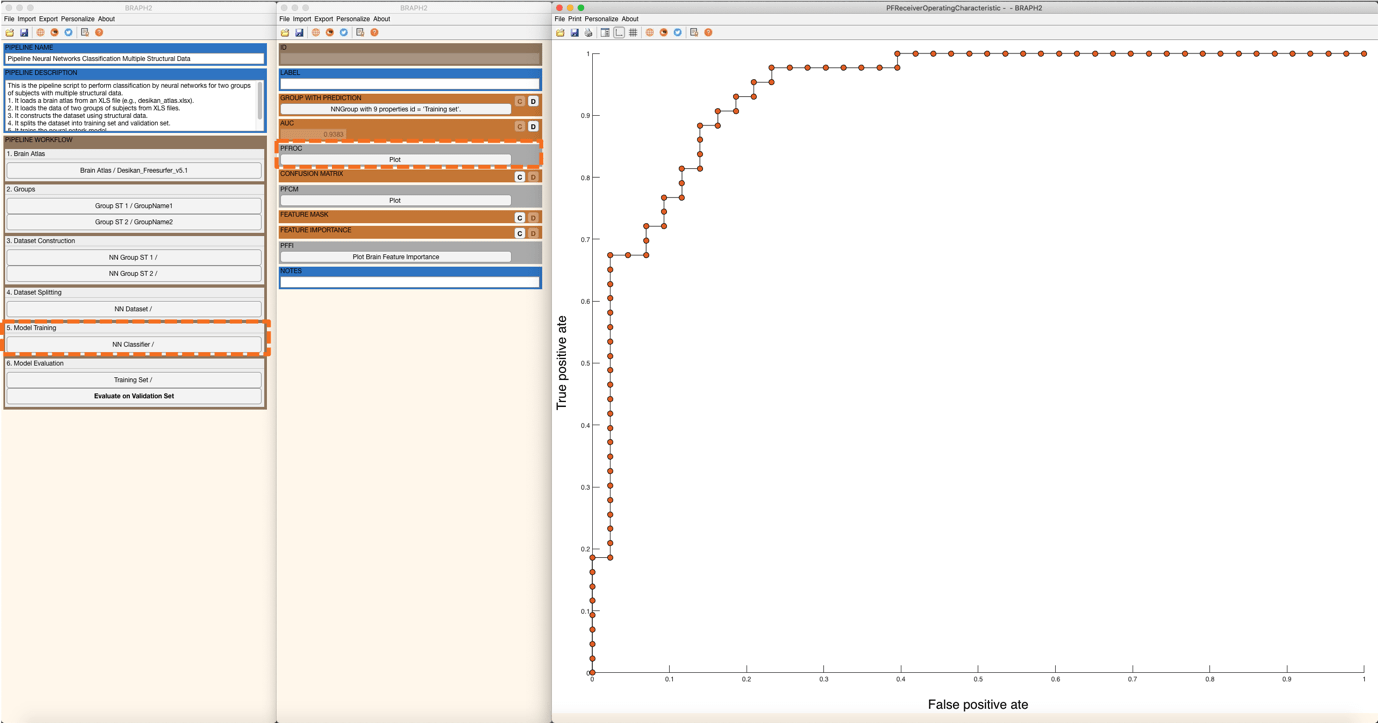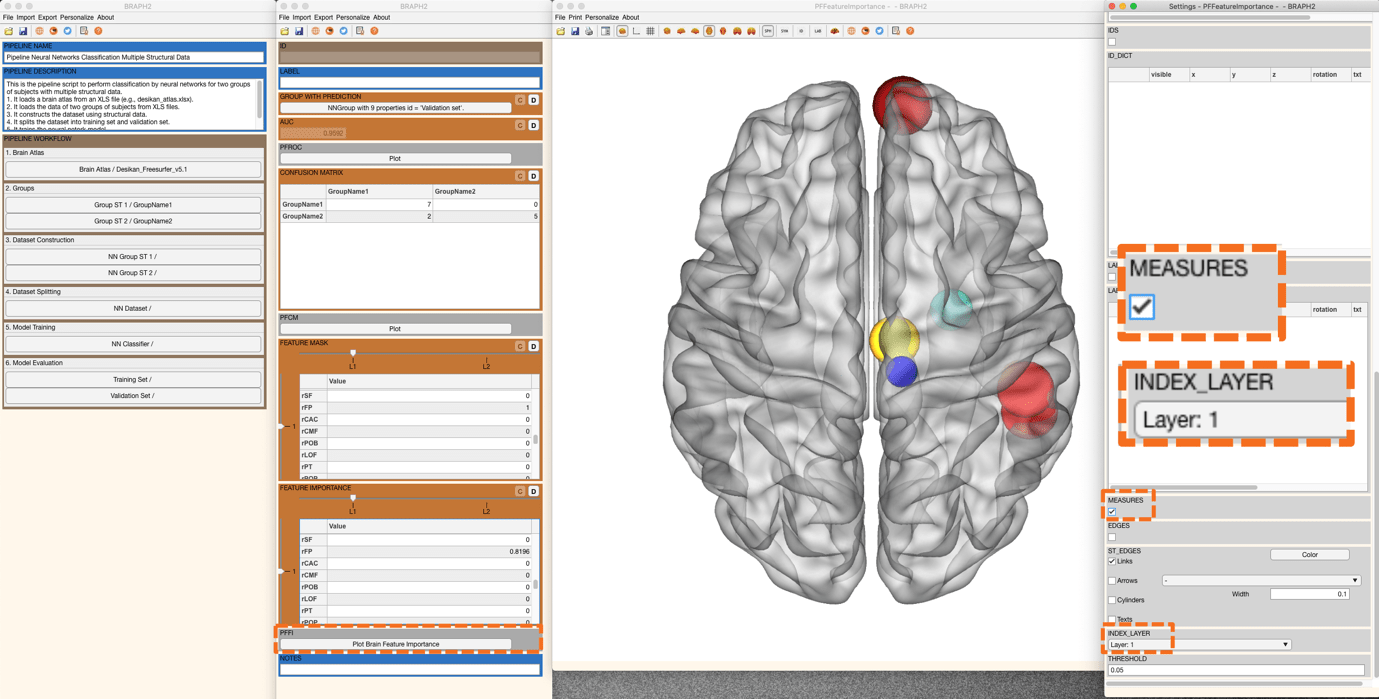# Pipeline Neural Networks Classification Multiple Structural Data

Description:

This tutorial is to demonstrate how to use the Graphical User Interface (GUI) to perform a classification using neural networks for two groups of subjects with their multiple structural data.

Each step will be illustrated with our example data. You can use your own data as well. See Tutorial_data_format_braph2 for how to prepare your structural data for BRAPH2.

• Start BRAPH 2 and select the Pipeline from the main GUI

Start MATLAB, navigate to the BRAPH2 folder and run “braph2” with the following command:

>> braph2

Select the Pipeline Neural Networks Classification Multiple Structural Data in the right menu. You can use the search field, typing Neural Networks Classification for example.

The first step is to load a brain atlas from an XLS file. Press the button “Load a Brain Atlas XLS” (the only one active in the pipeline GUI) and then select the desikan_atlas.xlsx in the directory ./braph2/pipelines/structural multiplex /example data ST_MP (MRI).

Finally, you can visualize the atlas by pressing “Plot the brain atlas” and playing with the surface settings. Check the Brain Atlas section from the tutorial of module 1 for more information on how to control the appearance of this interface.

To load the data for the two groups you would like to compare press “Load Group ST 1 from XLS” and select the folder for subjects of Group 1 which is at the directory ./braph2/pipelines/structural multiplex /example data ST_MP (MRI)/xls/Groupname1. After, load the data for Group 2 which can be found at the directory ./braph2/pipelines/structural multiplex /example data ST_MP (MRI)/xls /GroupName2.

• Dataset Construction

Overall, this step decides the input for running the classification. Here, we use the structural data from the selected group.

Press “NN Dataset for Group 1” from the main pipeline GUI. For INPUT TYPE, select structural_data (this is the only option when using structural data). For TARGET NAME, it is set according to the folder name where this group is from (Figure 1). After setting up INPUT TYPE and TARGET NAME, press “C” to create the dataset and move on to the next step.

For group 2, press “NN Dataset for Group 2” in the main GUI and similarly obtain the dataset.Figure 1. Constructing the dataset.

• Dataset Splitting

This step splits the data into a training set and a validation set.

Press “Split the Dataset” in the main pipeline GUI and an interface will be prompted for setting up the split. You can specify the index of the subjects who are assigned to the validation set (e.g., [2 3 5 7], meaning subject 2, subject 3, subject 5, and subject 7) or alternatively, you can type a number (between 0 and 1) that indicates the proportion of the subjects who are assigned to the validation set (e.g., 0.5, meaning half of the subject will be assigned to the validation set) (Figure 2). Here, we set to 0.15 the proportion of validation set in both groups. Finally, we press “C” in TRAINING GROUP and after “C” in VALIDATION GROUP to create the datasets.Figure 2. Splitting the dataset into the training set and validation set.

• Model Training

This step will train the neural network model with the specified parameters.

Press “Train the NN” in the main pipeline GUI and an interface will be prompted to set the options/parameters (LAYERS, BATCH, EPOCHS, SHUFFLE, SOLVER, FEATURE SELECTION PROPORTION, VERBOSE, PLOT TRAINING PROGRESS, PLOT LAYERS) for training neural networks.

Descriptions for each option/parameter are illustrated below:

LAYERS: This parameter specifies (i) how many fully connected layers are there and (ii) how many neurons are there in each layer as a vector. For instance, “[100 50]” means that there are two layers: 100 neurons for the first layer, and 50 neurons for the second layer.

(Note. In this case, each fully connected layer is followed by a dropout layer with a given dropout rate of 0.5.)

BATCH: This parameter specifies the size of the batch for each training iteration (as a positive integer). A batch is a subset of the training set and is used to (i) evaluate the gradient of the loss function and (ii) update the weights.

EPOCHS: This parameter specifies the maximum number of epochs for training (as a positive integer). An epoch is the full pass of the training algorithm over the entire training set.

SHUFFLE: This parameter specifies the option for data shuffling. There are three options: “once”, “never”, and “every-epoch”. “once” means to shuffle the training and validation data once before training. “Never” means not to shuffle the data at all. “Every-epoch” means to shuffle the training data before each training epoch.

SOLVER: This parameter specifies the option of solver. There are three options: “sgdm”, “rmsprop”, and “adam”. “sgdm” uses the stochastic gradient descent with momentum (SGDM) optimizer. “rmsprop” uses the RMSProp optimizer. “adam” uses the Adam optimizer.

FEATURE SELECTION PROPORTION: This parameter specifies the proportion of the features to be selected for training the neural networks. All the features are analyzed and given individual scores based on the mutual information analysis. Based on the scores, all the features can be ranked, and the user can set a proportion for including part of the ranked features that are relatively informative.

VERBOSE: This parameter indicates whether to display training progress information in the command window.

PLOT TRAINING PROGRESS: This parameter indicates whether to create a figure and displays training metrics at every iteration.

PLOT LAYERS: This parameter indicates whether to create a figure to display the neural network architecture.Figure 3. Setting up the parameters for training neural networks.

After changing the FEATURE SELECTION PROPORTION to 0.1 and checking VERBOSE, you can now train the model by clicking on the “C” in MODEL. In this example, you will see the verbose in the command line window (Figure 3).

• Model Evaluation

In this step, we evaluate the performance of the neural network model on the training set as well as on the validation test.

After training the model we evaluate its performance. First, we evaluate the performance of the training set by pressing “Evaluate on Training Set” in the main pipeline GUI. An interface will open, and we can calculate GROUP WITH NN PREDICTION, AUC (Figure 4), and CONFUSION MATRIX (Figure 5).

In GROUP WITH NN PREDICTION, one can get a group in which subjects convey the prediction from the trained neural networks.

In AUC, one can get the area under the receiver operating characteristic, a measure to evaluate the model’s performance when compared to actual target values. We can also plot the ROC curve by pressing PFROC (Figure 4). You can also adjust the figure with the settings panel (Figure 4).Figure 4. Plot of receiver operating characteristic curve.

In CONFUSION MATRIX, one can get the confusion matrix determined by the target and predicted groups. Press the plot button in PFCM to plot the confusion matrix (Figure 5).Figure 5. Confusion matrix.

Now, it is time to calculate the feature mask. The Feature Mask indicates which features are selected to train/test the model. More interestingly, we can calculate the Feature Importance (Figure 6). After pressing the “C” in FEATURE IMPORTANCE to calculate the feature importance, we can proceed to visualize it on a brain surface. For that, you can right-click on “Plot Brain Feature Importance”.Figure 6. Visualization of the feature importance for layer 1.

A new figure with the brain surface will be prompted for brain region visualization (all with default settings). Go to settings and scroll down in the Settings panel. You will find Measure and please tick on the box. Now, the color and size of all spheres will be adapted in proportion to the feature importance’s value, where blueish colors represent smaller values, and red larger (Figure 6). By default, we will see the feature importance of the first layer.

Note that, since we have two layers of input data, by switching INDEX_LAYER, we can change the visualization of feature importance from one layer to the other. We explore also the feature importance in layer 2 (Figure 7)Figure 7. Visualization of the feature importance for layer 2.

Finally, we evaluate the performance on the validation set, by pressing “Evaluate on Validation Set” in the main pipeline GUI and similarly to the evaluation on the training set, we can obtain the AUC, CONFUSION MATRIX, FEATURE MASK, and FEATURE IMPORTANCE (Figure 8).Figure 8. Visualization of the feature importance for the validation set.